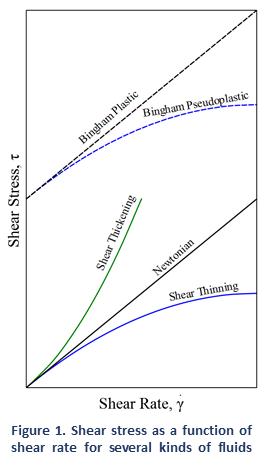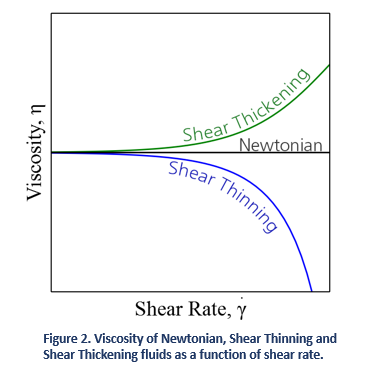# Viscosity of Newtonian and non-Newtonian Fluids

Successful characterization of viscosity is key in determining if a fluid is Newtonian or non-Newtonian

## Viscosity of Newtonian and non-Newtonian Fluids

If you are on this site, you probably have a general idea about what is viscosity and how important it is in the development of any application that involves fluid flow. However, fluid characterization is far more deep and complex than what is usually expected. Each unique material has its own behavior when subjected to flow, deformation or stress.

Depending on their viscosity behavior as a function of shear rate, stress, deformation history..., fluids are characterized as Newtonian or non-Newtonian.

### Newtonian Fluids

Newtonian fluids are named after Sir Issac Newton (1642 - 1726) who described the flow behavior of fluids with a simple linear relation between shear stress [mPa] and shear rate [1/s]. This relationship is now known as Newton's Law of Viscosity, where the proportionality constant η is the viscosity [mPa-s] of the fluid:Some examples of Newtonian fluids include water, organic solvents, and honey. For those fluids viscosity is only dependent on temperature. As a result, if we look at a plot of shear stress versus shear rate (See Figure 1) we can see a linear increase in stress with increasing shear rates, where the slope is given by the viscosity of the fluid. This means that the viscosity of Newtonian fluids will remain a constant (see Figure 2) no matter how fast they are forced to flow through a pipe or channel (i.e. viscosity is independent of the rate of shear).

An exception to the rule is Bingham plastics, which are fluids that require a minimum stress to be applied before they flow. These are strictly non-Newtonian, but once the flow starts they behave essentially as Newtonian fluids (i.e. shear stress is linear with shear rate). A great example of this kind of behavior is mayonnaise.

Newtonian fluids are normally comprised of small isotropic (symmetric in shape and properties) molecules that are not oriented by flow. However, it is also possible to have Newtonian behavior with large anisotropic molecules. For example, low concentration  protein or polymer solutions might display a constant viscosity regardless of shear rate. It is also possible for some samples to display Newtonian behavior at low shear rates with a plateau known as the zero shear viscosity region.

### Non-Newtonian FluidsIn reality most fluids are non-Newtonian, which means that their viscosity is dependent on shear rate (Shear Thinning or Thickening) or the deformation history (Thixotropic fluids). In contrast to Newtonian fluids, non-Newtonian fluids display either a non-linear relation between shear stress and shear rate (see Figure 1), have a yield stress, or viscosity that is dependent on time or deformation history (or a combination of all the above!).

A fluid is shear thickening if the viscosity of the fluid increases as the shear rate increases (see Figure 2). A common example of shear thickening fluids is a mixture of cornstarch and water. You have probably seen examples of this on TV or the internet, where people can run over this kind of solutions and yet, they will sink if they stand still.  Fluids are shear thinning if the viscosity decreases as the shear rate increases. Shear thinning fluids, also known as pseudo-plastics, are ubiquitous in industrial and biological processes. Common examples include ketchup, paints and blood.

Non-Newtonian behavior of fluids can be caused by several factors, all of them related to structural reorganization of the fluid molecules due to flow. In polymer melts and solutions, it is the alignment of the highly anisotropic chains what results in a decreased viscosity. In colloids, it is the segregation of the different phases in the flow that causes a shear thinning behavior.

### Why Should I Care?Fluid flow is highly dependent on the viscosity of fluids. At the same time for a non-Newtonian fluid, the viscosity is determined by the flow characteristics . Looking at Figure 3, you can observe three very different velocity profiles depending on the fluid behavior. For all these fluids, the shear rate at the walls (i.e. the slope of the velocity profile near the wall) is going to determine viscosity. Successful characterization of viscosity is key in determining if a fluid is Newtonian or non-Newtonian, and what range of shear rates needs to be considered for an specific application. Many viscometers on the market measure index viscosity but often lack proper characterization of shear rate and absolute or true viscosity. Absolute viscosity is one of the most important parameters in the development and modeling of applications that involve fluid flow. Therefore, proper characterization of viscosity must be carried out at a shear rate that is relevant to the specific process.  Learn more about RheoSense viscometers and how they allow measurements of true viscosity over a wide range of shear rates.

### Newtonian & non-Newtonian WebinarWatch Webinar
Newtonian vs. non-Newtonian Fluids

Flow behavior of complex fluids is traditionally characterized through the distinction between Newtonian and non-Newtonian based on each fluid's viscosity dependence on the rate of deformation and the rate of shear rate. Proper understanding of rheological properties such as shear stress, shear strain, and shear rate are vital in practical applications such as inkjet printingprotein formulations/injections, and food/beverage manufacturing.

RheoSense’s webinar explains the differences between Newtonian and non-Newtonian fluids and covers the fundamentals of viscosity using real application examples.